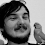# logji

noodling towards a functional brain

## Tuesday, December 08, 2009

```trait A {
def accept[T](v: V[T]): T
}

class B(val s: String) extends A {
var i = 0
override def accept[T](v: V[T]): T = v.visit(this)
}

class C(val s: String) extends A {
var i = 0
override def accept[T](v: V[T]): T = v.visit(this)
}

class D(val s: String) extends A {
var i = 0
override def accept[T](v: V[T]): T = v.visit(this)
}

trait V[T] {
outer =>
def visit(b: B): T
def visit(c: C): T
def visit(d: D): T

def map[U](f: T => U): V[U] = new V[U] {
def visit(b: B): U = f(outer.visit(b))
def visit(c: C): U = f(outer.visit(c))
def visit(d: D): U = f(outer.visit(d))
}

def flatMap[U](f: T => V[U]): V[U]= new V[U] {
def visit(b: B): U = f(outer.visit(b)).visit(b)
def visit(c: C): U = f(outer.visit(c)).visit(c)
def visit(d: D): U = f(outer.visit(d)).visit(d)
}
}

object V {
def pure[T](t: T): V[T] = new V[T] {
def visit(b: B) = t
def visit(c: C) = t
def visit(d: D) = t
}
}
```
And the test:
```object Test {
def main(argv: Array[String]) {
class VI(i: Int) extends V[Int] {
def visit(b: B) = error("not supported")

def visit(c: C) = {
println("vi: " + c.s + " = " + c.i)
c.i = c.i + i
c.i
}

def visit(d: D) = {
println("vi: " + d.s + " = " + d.i)
d.i = d.i + i
d.i
}
}

def f(i: Int): V[Int] = {
println("f: " + i)
new VI(i)
}

def g(i: Int): V[Int] = {
println("g: " + i)
new VI(i)
}

val c1: A = new C("c1")
val c2: A = new C("c2")
val d1: A = new D("d1")
val v = new VI(1)
println(c1.accept(v.flatMap(f).flatMap(g)))
println(c2.accept(v.flatMap(x => f(x).flatMap(g))))
println(d1.accept(v.flatMap(f).flatMap(g).flatMap(f).flatMap(g)))
}
}
```
As far as I can tell, this satisfies the monad laws. Am I missing anything? If not, this is the first monad I've "found in the wild!"

1.This is amazingly beautiful. Thanks a lot!

2.4 years late, but isn't this exactly the free monad over the F-algebra exposed by your visitor?

3.9 years later, rereading; seems like I want to add this to my book!